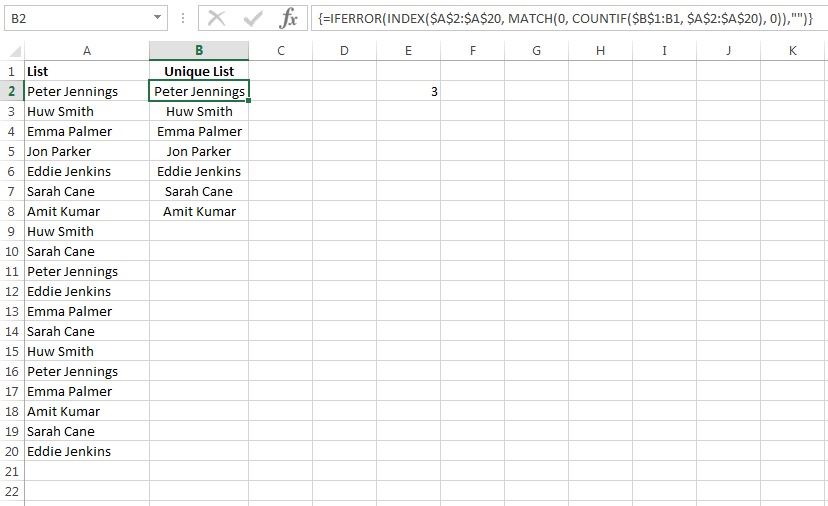Our Recent Posts

Archive

Tags

No tags yet.

# How to Extract a Unique List in Excel: Array Formula

I am often asked how you can extract a unique list of values from a data set containing duplicate values. A quick and easy way to extract a list of unique values from duplicate items is by creating a Pivot Table. The problem with this is that whenever you have new data you have to keep on refreshing the Pivot Table. What about if you want the list to be dynamic, i.e. the unique list will update automatically whenever there is new data? Well the best way to do this is by creating an array formula.

I will teach you how to create the array formula and then break the formula down so you know how it is calculated.

### Array Formula to Extract Unique ValuesIn column A there is a list of names. As you can see the names have been repeated. I want to create a unique list in column B so there are no duplicate entries.The formula in cell B2 is ={INDEX(\$A\$2:\$A\$20, MATCH(0, COUNTIF(\$B\$1:B1, \$A\$2:\$A\$20), 0))}. This is an array formula so you must press Control + Shift + Enter after you have typed in the formula. Once I have created the formula in cell B2 I just copied it down to cell B20. You can see that the formula has created a unique list of names. Duplicate names are not included.

You will notice the #N/A errors. This occurs when all the unique names have been extracted and there are no more names left to extract.To eliminate these #N/A errors you can wrap the array formula in an IFERROR function.

### How does the Array Formula Work?

The array formula contains the COUNTIF, MATCH, INDEX and IFERROR functions. I will use the formula in cell B2 to explain how it works:

1) The COUNTIF Function

COUNTIF(\$B\$1:B1, \$A\$2:\$A\$20)

The COUNTIF function counts the number of cells that meet a criteria in a given range.

The COUNTIF function in cell B2 will return:

COUNTIF("Unique distinct list",{Peter Jennings, Huw Smith, Emma Palmer, Jon Parker, Eddie Jenkins, Sarah Cane, Amit Kumar, Huw Smith, Sarah Cane, Peter Jennings, Eddie Jenkins, Emma Palmer, Sarah Cane, Huw Smith, Peter Jennings, Emma Palmer, Amit Kumar, Sarah Cane, Eddie Jenkins})

This will return an array which will contain 1 or 0 based on if the range B\$1:B1 is found in the list in \$A\$2:\$A\$20.

The COUNTIF function will return the result array {0;0;0;0;0;0;0;0;0;0;0;0;0;0;0;0;0;0;0} as \$B\$1:B1 (“Unique List”) is not found in the range \$A\$2:\$A\$20

2) The MATCH Function

MATCH(0, COUNTIF(\$B\$1:B4, \$A\$2:\$A\$20), 0)

The MATCH function returns the position of the lookup value in an array.

The MATCH function in cell B2 will return the array:

MATCH(0,{0;0;0;0;0;0;0;0;0;0;0;0;0;0;0;0;0;0;0},0)

The lookup value is 0 and the first value in the result array is 0 so the function returns 1.

3) The INDEX Function

INDEX(\$A\$2:\$A\$20, MATCH(0, COUNTIF(\$B\$1:B4, \$A\$2:\$A\$20), 0))

The INDEX function returns the cell value based on the row number specified. The MATCH function returns the row number 1 as explained above so the INDEX function uses the first value in the range \$A\$2:\$A\$20 and returns "Peter Jennings".

4) The IFERROR Function

IFERROR(INDEX(\$A\$2:\$A\$20, MATCH(0, COUNTIF(\$B\$1:B1, \$A\$2:\$A\$20), 0)),"")

The IFERROR function removes all the #N/A errors and returns an empty string instead.

Relative and Absolute Cell References

Notice the relative cell referencing on \$B\$1:B1 and absolute referencing on the range \$A\$2:\$A\$20. This is because we want to include the names in the formula in column B to check how many there are in column A as we copy it down. In order for the formulas to work correctly we also have to make sure the range A2:A20 remains constant as we copy the formula down.

### Conclusion

I hope you enjoyed this tutorial on how to extract a unique list in Excel from a list of duplicate values. Please leave a comment below if you would like a further explanation or help with the array formula or if you know of any other ways to extract a unique list.